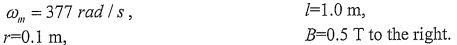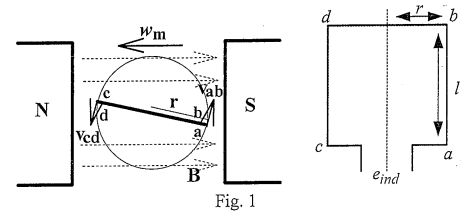lvy>試卷(2022/08/05)

# 103 年 - 103 國立中山大學_碩士班招生考試_電機系(丁組)：電力工程#110072

【非選題】
1.

1. Fig. 1 shows a simple loop rotating in a uniform magnetic field. It has the following characteristics (25%):Suppose that a 5Ω resistor is connected as a load across the terminals of the loop.【題組】 (a) Calculate the voltage induced in the rotating loop () and the current flowing through the resistor. (10%)

【非選題】
2.【題組】(b) Calculate the induced torque, electric power being generated and mechanical power being consumed by the loop. (15%)

【非選題】
3.

2. Calculate the geometric mean radius of conductors shown in Fig. 2 in terms of the radius r of an individual strand. (10%)【非選題】
4.3. Discuss the solution procedures for a power flow problem solved by a Netwon-Raphson technique. (including the procedure for swing bus, PV bus and PQ bus) (15%)

【非選題】
5.

4. The impedance matrix () of a power system isCalculate the short-circuit current for a three-phase fault occurred in bus 2 and determine the bus voltages during the fault (15%).

【非選題】
6.

5. Derive the solution for the following economic dispatch problem by Lagrangian multiplier (15%)【非選題】
7.

6.Assume that the impedances for zero-sequence, positive-sequence and negative-sequence networks are Z0, Z1 and Z2 , respectively. Derive the formulas for I0, I1 , I2, and Ia and draw the interconnected sequence networks for a single line-to-ground fault as shown in Fig. 3 (20%)...